# PSEB 5th Class Maths Solutions Chapter 2 Fundamental Operations on Numbers Ex 2.7

Punjab State Board PSEB 5th Class Maths Book Solutions Chapter 2 Fundamental Operations on Numbers Ex 2.7 Textbook Exercise Questions and Answers.

## PSEB Solutions for Class 5 Maths Chapter 2 Fundamental Operations on Numbers Ex 2.7

Question 1.
Solve the following :
(a) 117 ÷ 13
(b) 135 ÷ 15
(c) 72 ÷ 12
(d) 108 ÷ 9
(e) 78 ÷ 13
(f) 121 ÷ 11
(g) 140 ÷ 20
(h) 144 ÷ 16
(i) 98 ÷ 14
(j) 119 ÷ 17.
Solution: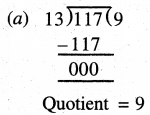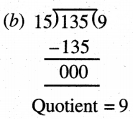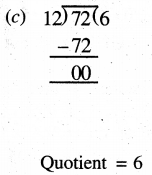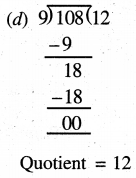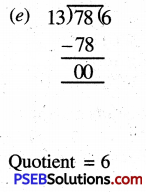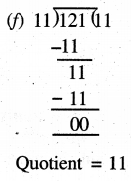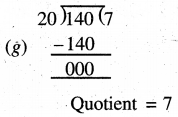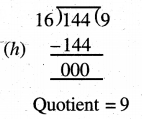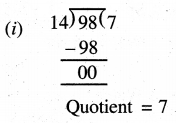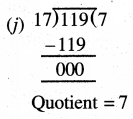Question 2.
Divide the following and verify :
(a) 54598 ÷ 12 .
(b) 8975 ÷ 21
(c) 77552 ÷ 18
(d) 88001 ÷ 17
(e) 12896 ÷ 11.
Solution: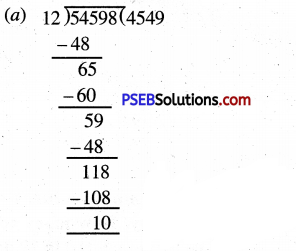Quotient = 4549
Remainder = 10
Verification :
Dividend = Quotient × Divisor + Remainder
54598 = 4549 × 12 + 10
54598 = 54588 + 10
54598 = 54598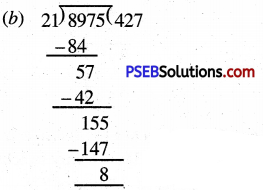Quotient = 427
Remainder = 8
Verification :
Dividend = Quotient × Divisor + Remainder
8975 = 427 × 21 + 8
8975 = 8967 + 8
8975 = 8975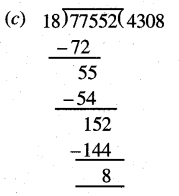Quotient = 4308
Remainder = 8
Verification :
Dividend = Quotient × Divisor + Remainder
77552 = 4308 × 18 + 8
77552 = 77544 + 8
77552 = 77552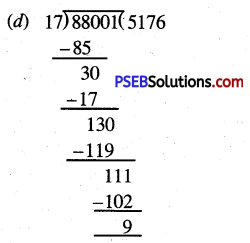Quotient = 5176
Remainder = 9
Verification :
Dividend = Quotient × Divisor + Remainder
88001 = 5176 × 17 + 9
88001 = 87992 + 9
88001 = 88001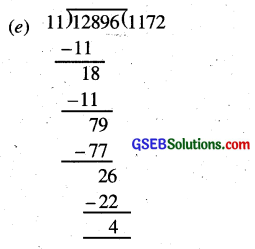Quotient = 1172
Remainder = 4
Verification :
Dividend = Quotient × Divisor + Remainder
12896 = 1172 × 11 + 4
12896 = 12892 + 4
12896 = 12896Question 3.
Solve the following and verify :
(a) 760 ÷ 12
(b) 550 ÷ 14
(c) 894 ÷ 21
(d) 913 ÷ 19
(e) 826 ÷ 25
(f) 7645 ÷ 24
(g) 89781 ÷ 9
(h) 99999 ÷ 80
(i) 82525 ÷ 75
(j) 70008 ÷ 14
(k) 50205 ÷ 15
(l) 16258 ÷ 36
(m) 96000 ÷ 50
(n) 45457 ÷ 35
Solution: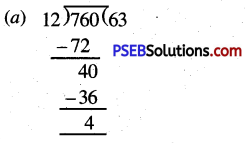Quotient = 63
Remainder = 4
Verification :
Dividend = Quotient × Divisor + Remainder
760 = 63 × 12 + 4
760 = 756 + 4
760 = 760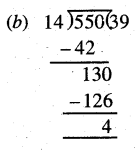Quotient = 39
Remainder = 4
Verification :
Dividend = Quotient × Divisor + Remainder
550= 39 × 14 + 4
550 = 546 + 4
550 = 550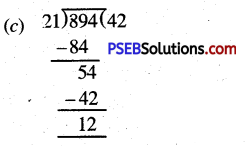Quotient = 42
Remainder = 12
Verification :
Dividend = Quotient × Divisor + Remainder
894 = 42 × 21 + 12
894 = 882 + 12
894 = 894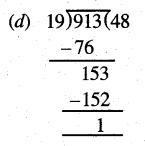Quotient = 48
Remainder = 1
Verification :
Dividend = Quotient × Divisor + Remainder
913 = 48 × 19 + 1
913 = 912 + 1
913 = 913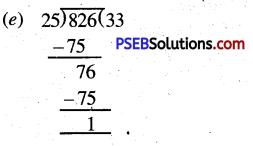Quotient = 33
Remainder = 1
Verification :
Dividend = Quotient × Divisor + Remainder
826 = 33 × 25 + 1
826 = 825 + 1
826 = 826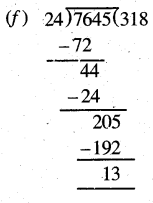Quotient = 318
Remainder = 13
Verification :
Dividend = Quotient × Divisor + Remainder
7645 = 318 × 24 + 13
7645 = 7632 + 13 .
7645 = 7645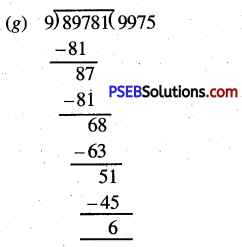Verification :
Dividend = Quotient × Divisor + Remainder
89781 = 9975 × 9 + 6
89781 = 89775 + 6 89781 = 89781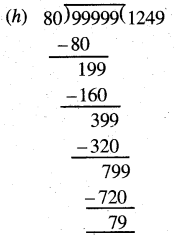Quotient = 1249
Remainder = 79
Verification :
Dividend = Quotient × Divisor + Remainder
99999 = 1249 × 80 + 79
99999 = 99920 + 79
99999 = 99999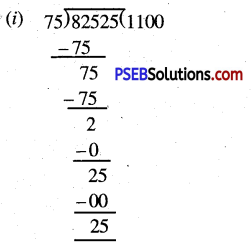Quotient = 1100
Remainder = 28
Verification :
Dividend = Quotient × Divisor + Remainder
82525 = 1100 × 75 + 25
82525 = 82500 + 25
82525 = 82525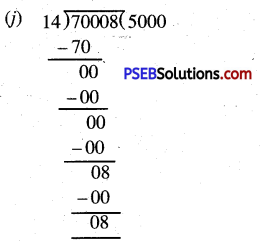Quotient = 5000
Remainder = 8
Verification :
Dividend = Quotient × Divisor + Remainder
70008 = 5000 × 14 + 08
70008 = 70000 + 08
70008 = 70008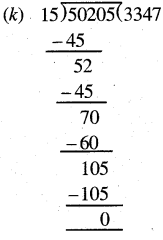Quotient = 3347
Remainder = 0
Verification :
Dividend = Quotient × Divisor + Remainder
50205 = 3347 × 15 + 0
50205 = 50205Quotient = 451
Remainder = 22
Verification :
Dividend = Quotient × Divisor + Remainder
16258 = 451 × 36 + 22
16258 = 16258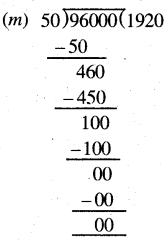Quotient = 1920
Remainder = 0
Verification :
Dividend = Quotient × Divisor + Remainder
96000 = 1920 × 50 + 0
96000 = 96000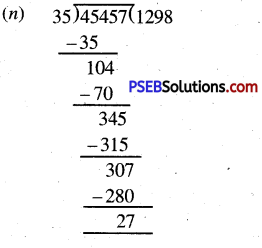Quotient = 1298
Remainder = 27
Verification :
Dividend = Quotient × Divisor + Remainder
45457= 1298 × 35 + 27
45457 = 45430 + 27
45457 = 45457